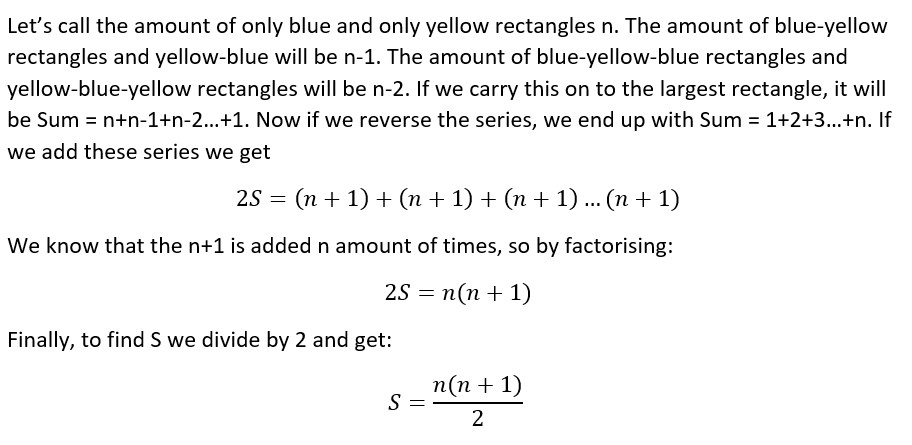#### You may also like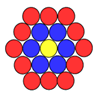### Counting Counters

Take a counter and surround it by a ring of other counters that MUST touch two others. How many are needed?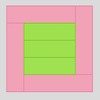### Cuisenaire Squares

These squares have been made from Cuisenaire rods. Can you describe the pattern? What would the next square look like?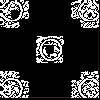### Doplication

We can arrange dots in a similar way to the 5 on a dice and they usually sit quite well into a rectangular shape. How many altogether in this 3 by 5? What happens for other sizes?

# Counting Stick Conjectures

##### Age 5 to 11Challenge Level

Well done to everybody who investigated the number of rectangles inside a counting stick. We received a lot of solutions to this activity and unfortunately we don't have space for all of them here. Thank you to all the children at Highgate Junior School in the UK who sent in their ideas, and thank you as well to Ryan from Kong Hwa School in Singapore, Lawrence from YCIS in China, and Amy and Teija from Pierrepont Gamston Primary School in England. You all had some great suggestions similar to the ones we've featured below.

Melissa from Coalway Junior in the UK approached this task systematically. She said:

The amount of 1 blocks you can fit in it is 10.

The amount of 2 blocks you can fit in it is 9.

The amount of 3 blocks you can fit in it is 8.

The amount of 4 blocks you can fit in it is 7.

The amount of 5 blocks you can fit in it is 6.

The amount of 6 blocks you can fit in it is 5.

The amount of 7 blocks you can fit in it is 4.

The amount of 8 blocks you can fit in it is 3.

The amount of 9 blocks you can fit in it is 2.

The amount of 10 blocks you can fit in it is 1.

There are 55 rectangles.

Well done, Melissa! How did you work out that this makes 55 rectangles altogether? Is there a quick way of adding up all those numbers?

Marie and Sophia from Phorms München in Germany used a very similar method, and sent in this picture to demonstrate: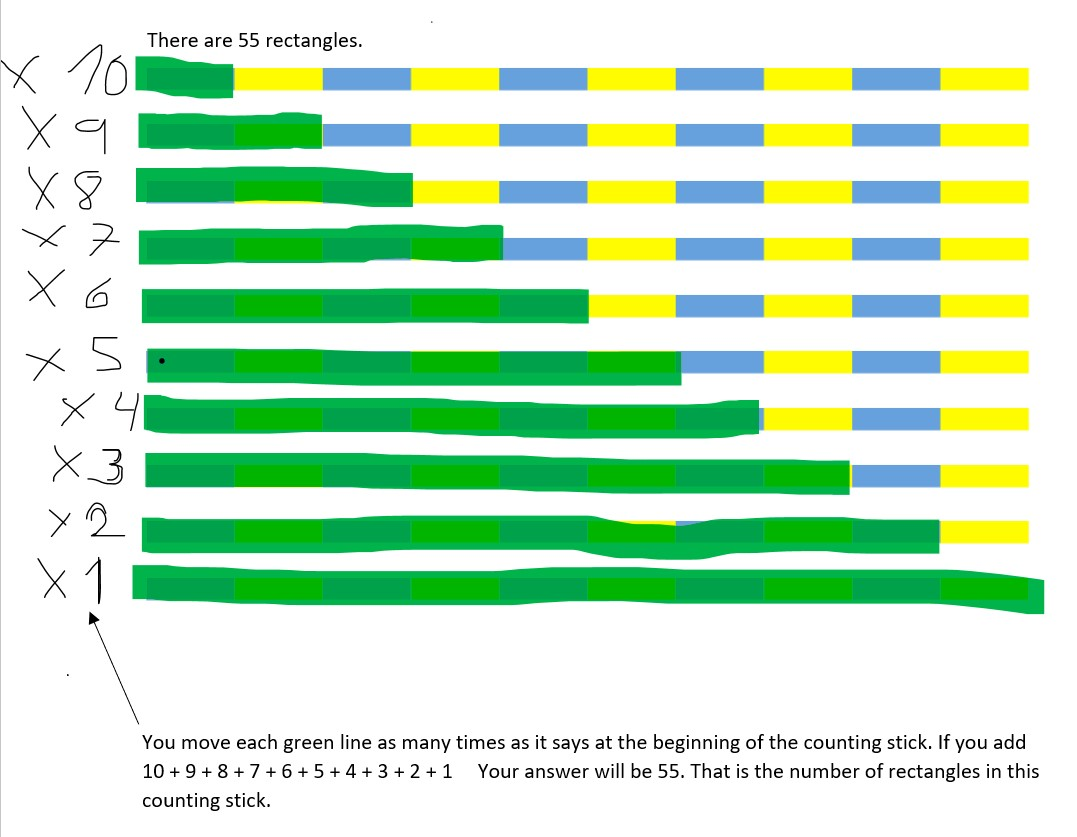Thank you both for sending in that very clear picture.

Luca from Highgate Junior School in the UK thought about this in a different way. Have a look at Luca's explanation and see if you can follow his method: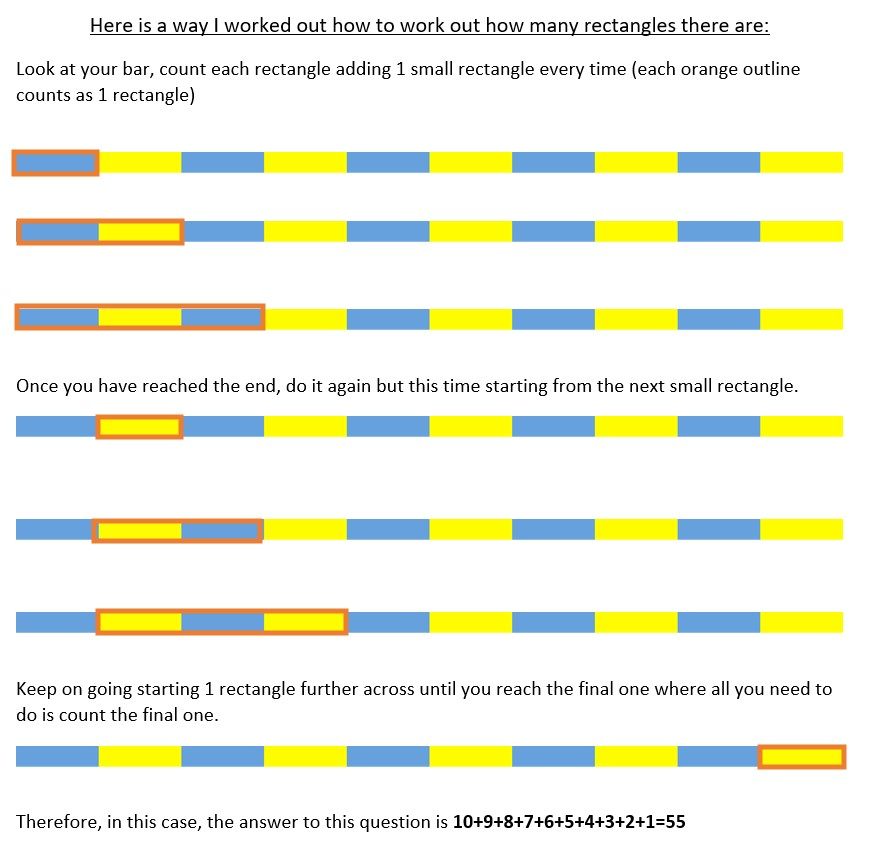Well done Luca! Luca found all of the rectangles that started with the first small rectangle, then all of the rectangles that started with the second, all the way up to the rectangles that start with the final small rectangle (which will just be one rectangle). I wonder if we can expand Luca's method to find a way of counting all the rectangles inside a counting stick of any size?

Sophia from Highgate had a think about the general pattern. She said:

At first I looked at the problem and thought there were 10 rectangles (all the different coloured small rectangles). I soon realised that there would be many rectangles inside the larger rectangle and the larger rectangle as well so there would be a lot more than 10. I started by focusing on the first rectangle there was only one. I then went on to the second rectangle. I realised that there would be one rectangle that was the first two squares and a rectangle which was just the second rectangle. I then came up with a theory, my theory is that whatever rectangle you are on the rectangles that fit inside the rectangles before and the rectangle you are on would add up to how may rectangles would fit inside the current one. For example, take the fourth rectangle you would start by adding how many rectangles fit inside the first rectangle. Only one. You would then take the take the second rectangle. 2 new rectangles fit inside the second rectangle. If you add them together and then add them on to 3 since that is how many new rectangles fit inside the third rectangle you get 6. 6 rectangles fit inside the third rectangle. You then add this in to 4 since in the fourth rectangle you get 4 new rectangles. If you add 6 and 4 you get 10. This means that 10 rectangles fit inside the fourth rectangle. This is the formula to solve a problem like this. This would work all the way up to the hundreds and thousands.

This is a really interesting way of thinking about it, Sophia. I agree that the pattern works, and I wonder if anybody can explain why it works. Why do we add four new rectangles when we move from the counting stick of length three to the counting stick of length four?

Lots of other children also noticed that there is a pattern when we look at the rectangles inside counting sticks of different lengths. Angel from Highgate said:

When investigating the sequence I noticed that the difference between the terms went up by one each time starting with +2 then plus 3 then plus 4 etc.

Well done, Angel - this is a different way of thinking about Sophia's pattern.

We received lots of solutions pointing out that starting at 1 and following the pattern +2 +3 +4 gives us the triangle numbers, so well done to everybody who spotted this. Dhruv from St. Anne's RC Primary School sent in this table of results: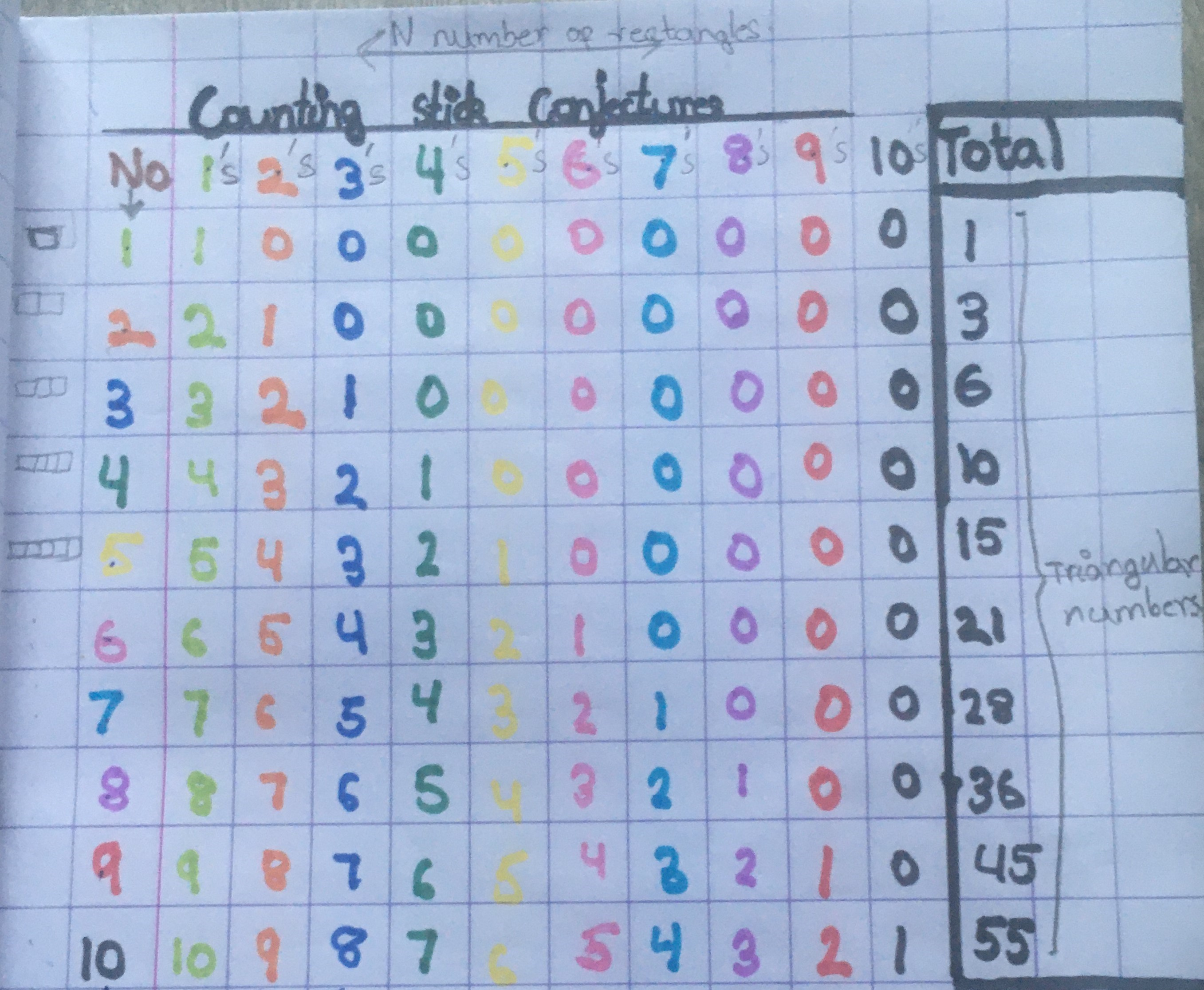This table makes the pattern very clear - well done!

Some children thought about quicker ways of adding the numbers of rectangles inside a counting square. Anya from Highgate noticed that the numbers in the sum 10 + 9 + 8 + 7 + 6 + 5 + 4 + 3 + 2 + 1 can be put into pairs that make 11. Have a look at Anya's full solution. I wonder what each pair of numbers might add up to if we change the length of the counting stick?

Oscar from Highgate used the fact that the pairs of numbers make 11 to find a quick way of adding up the numbers. He explained:

If I look at the entire ten box rectangle, there can only be one ten box rectangle. But if I look at nine box rectangles there are two possibilities: 1-9 and 2-10. If I look at 8 box rectangles there are three possibilities, 1-8, 2-9, 3-10.

I have worked out that each time I decrease the number of boxes (that form each rectangle) by one, the number of possibilities increases by one. The total number of possibilities is the sum of the numbers 1-10 because it's 1+2+3+4+5+6+7+8+9+10. The sum is 55.

An easy way to figure out the sum of a sequence of consecutive numbers is to add the first and last numbers and multiply the result by the number of pairs of numbers. Here this would be 1 plus 10 = 11 x 5 (pairs) = 55

Well done, Oscar. How do you know that we can just add the first and last numbers and multiply the result by the number of pairs?

Aran from Highgate explained why this method works. He said:

There is a formula that can be used to quickly find the number of rectangles in large sticks.

Starting with the stick with 6 blocks, I noticed that you could add the numbers in a different order

1+6 = 7

2+5 = 7

3+4 = 7

I.e.: (half of six) multiplied by (6+1) = 21 rectangles

This also works for all the other numbers!

Aran went on to describe a way that we can use algebra to write this method:

The formula is: If x = the number of small rectangles on the stick then x divided by 2 multiplied by (x+1) equals the number of possible rectangles. This formula works with both odd and even numbers.

So, working out how many rectangles are in a stick with 100 rectangles is a lot faster and easier now. All you need to do is use the formula. 100 divided by 2 x (100+1) = 5050 and a stick with 1000 small rectangles would just be 500,500 rectangles.

Well done, Aran! From your example, I'm convinced that the formula works for 6, but I'm not yet convinced that it definitely works for bigger numbers. We received lots of solutions saying that the formula was x divided by 2 multiplied by (x+1), but not many children could explain why it would always work.

Dhruv drew a picture to show how to add the numbers in pairs when finding the number of rectangles inside the 100-length counting stick: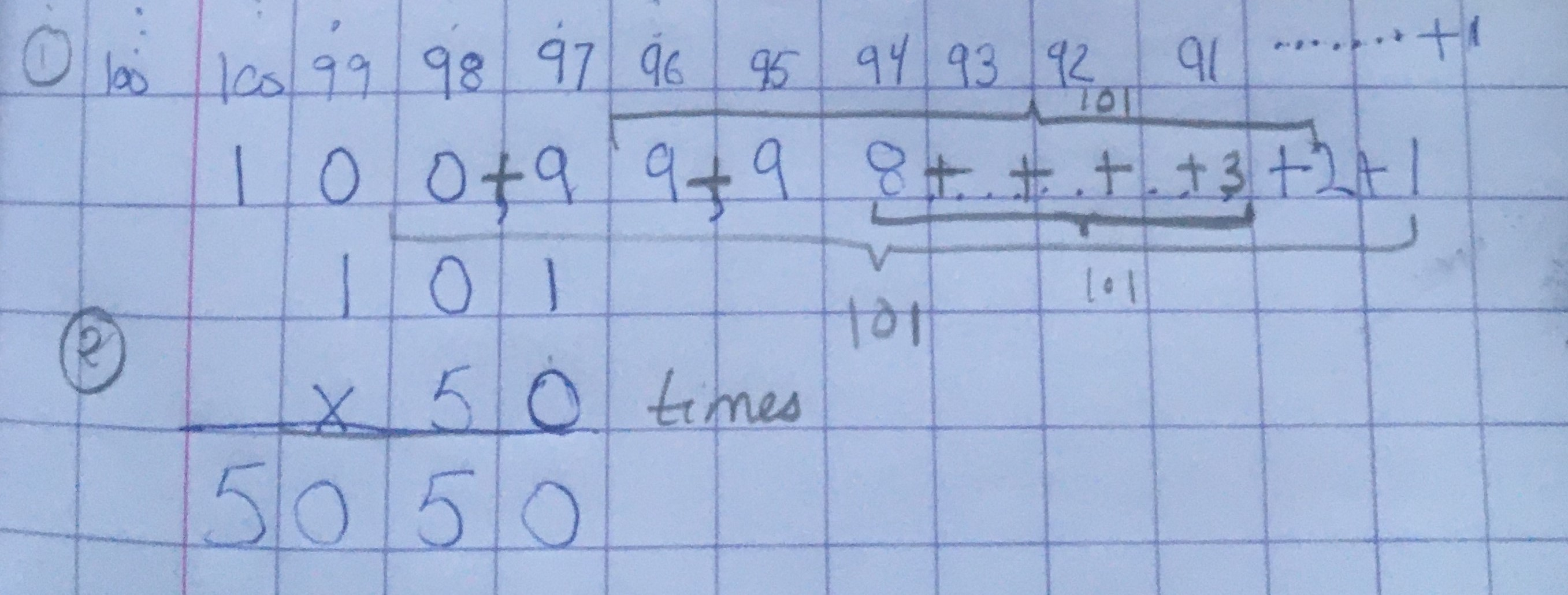Thank you for sending this in, Dhruv, it makes it very clear that we are adding 101 together lots of times. It's slightly trickier to check that we should be adding it together 50 times. I wonder if anybody can explain why it needs to be added 50 times?